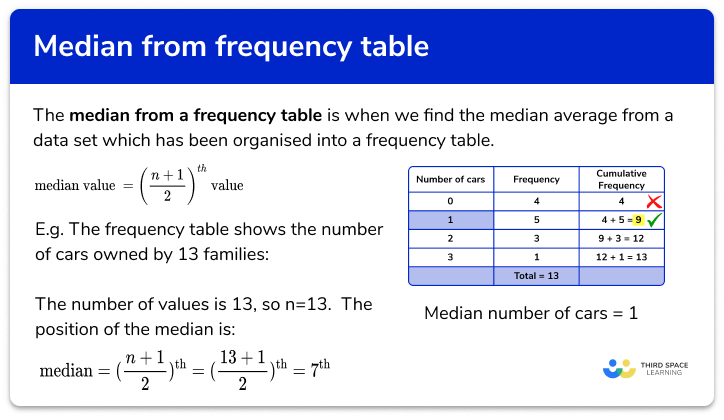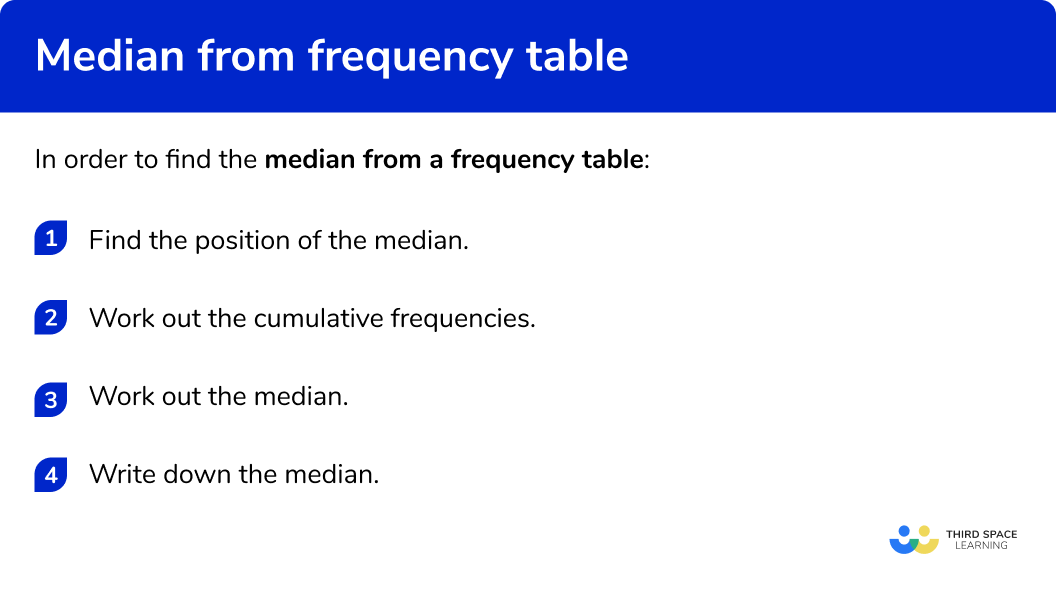GCSE Maths Statistics Frequency Tables

Median From a Frequency Table

# Median From A Frequency Table

Here we will learn about the median from a frequency table, including what the median is and how to find it.  We will also look at finding the class which contains the median from grouped frequency tables.

There are also averages from frequency tables worksheets based on Edexcel, AQA and OCR exam questions, along with further guidance on where to go next if you’re still stuck.

## What is median from a frequency table?

Median from a frequency table is when we find the median average from a data set which has been organised into a frequency table.

The median is the middle value and is a measure of central tendency, it is a value that can be used to represent a set of data.

We can calculate the median by:

a) Writing down each of the values in the table and crossing off values to find the middle value.

E.g.
The frequency table shows the number of cars owned by 13 families:

We can use the table to write down each of the values

The middle number is 1 , so the median number of cars is 1 .

b) Using the median formula and cumulative frequency

If the data set is large we can us the formula to work out the position of the median \text{Position of the median}=(\frac{n+1}{2})^\text{th} , and cumulative frequency to find the actual median value.

Cumulative frequency is where we add up the numbers in the frequency column as we go down the table.

E.g.

The total number of values is 13 , so n=13 .

So the, \text{Position of the median}=(\frac{n+1}{2})^\text{th}=(\frac{13+1}{2})^\text{th}=7^\text{th}

The 7^\text{th} value is in the row of the table that contains the 5^\text{th} , 6^\text{th} , 7^\text{th} , 8^\text{th} , and 9^\text{th} values.

So the median number of cars = 1 .

### What is median from a frequency table?## How to find the median from a frequency table

In order to find the median from a frequency table:

1. Find the position of the median.
2. Work out the cumulative frequencies.
3. Work out the median.
4. Write down the median.

### How to find the median from a frequency table## Related lessons on frequency tables

Median from a frequency table is part of our series of lessons to support revision on frequency tables. You may find it helpful to start with the main frequency table lesson for a summary of what to expect, or use the step by step guides below for further detail on individual topics. Other lessons in this series include:

## Median from a frequency table examples

### Example 1: frequency table – odd number of values

The frequency table shows the ages in years of 15 people in a sports club.

Find the median of age from this set of data.

1. Find the position of the median.

There are 15 values, so n=15 .

\text{Position of the median}=(\frac{n+1}{2})^\text{th}=(\frac{15+1}{2})^\text{th}=8^\text{th}

2Work out the cumulative frequencies.

Go down the frequencies, adding them up as you go along.

3Work out the median.

We use the cumulative frequencies to find where the 8^\text{th} value is. This row contains the 8^\text{th} to 14^\text{th} values.

4Write down the median.

The final step is to write down the median value

The median is 14 years

### Example 2: frequency table – odd number of values

The frequency table shows the temperature at midday for 17 days.

Find the median:

There are 17 values, so n=17 .

\text{Position of the median}=(\frac{n+1}{2})^\text{th}=(\frac{17+1}{2})^\text{th}=9^\text{th}

Go down the frequencies, adding them up as you go along.

We use the cumulative frequencies to find where the 9^\text{th} value is. This row contains the 9^\text{th} to 15^\text{th} values.

The final step is to write down the median value

The median is 24 °C

### Example 3: frequency table – even number of values

The frequency table shows the number of people in 12 train carriages.

Find the median:

There are 12 values, so n=12 .

\text{Position of the median}=(\frac{n+1}{2})^\text{th}=(\frac{12+1}{2})^\text{th}=6.5^\text{th}

We need to look for the 6^\text{th} and 7^\text{th} values.

Go down the frequencies, adding them up as you go along.

We use the cumulative frequencies to find where the 6^\text{th} and 7^\text{th} values are. This row contains the 3^\text{rd} to 7^\text{th} values.

The final step is to write down the median value

The median is 11 people

### Example 4: grouped data

The grouped frequency table shows the ages of 23 people at an event.

Find the class interval which contains the median:

There are 23 values, so n=23 .

\text{Position of the median}=(\frac{n+1}{2})^\text{th}=(\frac{23+1}{2})^\text{th}=12^\text{th}

We need to look for the 12^\text{th} value.

Go down the frequencies, adding them up as you go along.

We use the cumulative frequencies to find where the 12^\text{th} value is. This row contains the 10^\text{th} to 17^\text{th} values.

The final step is to write down the class interval which contains the median value

The median is in the class interval 10-14

### Example 5: grouped frequency table – odd number of values

The grouped frequency table shows the time spent by 21 students cleaning their rooms.

Find the class interval which contains the median:

There are 21 values, so n=21 .

\text{Position of the median}=(\frac{n+1}{2})^\text{th}=(\frac{21+1}{2})^\text{th}=11^\text{th}

We need to look for the 11^\text{th} value.

Go down the frequencies, adding them up as you go along.

We use the cumulative frequencies to find where the 11^\text{th} value is. This row contains the 9^\text{th} to 13^\text{th} values.

The final step is to write down the class interval which contains the median value

The median is in the class interval 10 to 19

### Example 6: grouped frequency table – even number of values

The grouped frequency table shows the amount of money 30 people spent in a shop.

Find the class interval which contains the median:

There are 30 values, so n=30 .

\text{Position of the median}=(\frac{n+1}{2})^\text{th}=(\frac{30+1}{2})^\text{th}=15.5^\text{th}

We need to look for the 15^\text{th} and 16^\text{th} values.

Go down the frequencies, adding them up as you go along.

We use the cumulative frequencies to find where the 15^\text{th} and 16^\text{th} values are. This row contains the 11^\text{th} to 19^\text{th} values.

The final step is to write down the class interval which contains the median value

The median is in the class interval 30\le x < 40

### Common misconceptions

• Check which average you are being asked for

Check if you have been asked for the median, mode or mean average.

• Position of the median

The value given by the median formula is not for the median, but for the position of the median. The formula gives us where the middle value is.

• Even number of items

When there is an odd number of data there is a single middle value for the median.
When there is an even number of data, there is a middle pair of values and you then need to find the midpoint of these values.

E.g.
The middle pair of values is 9 and 13 . The mid-point of these is 11 . The median is 11 .

\frac{9+13}{2}=\frac{22}{2}=11

• Class intervals notation

The class intervals used in grouped frequency tables can be written in different ways. Take care when writing them, or finding the midpoints.

E.g.

0 to 5

0-5

0\le x<5

• Frequency distribution table

A frequency distribution table is the same as a frequency table

### Practice median from a frequency table questions

1. The frequency table shows the number of pets of 15 students.
Find the median:21710We need to find the position of the median. There are 15 values, so n=15 .

\text{Position of the median}=(\frac{n+1}{2})^\text{th}=(\frac{15+1}{2})^\text{th}=8^\text{th}

We need the 8^\text{th} value.

This row contains the 4^\text{th} to 10^\text{th} values.2. The frequency table shows the ages of children in a drama club.
Find the median:981221We need to find the position of the median. There are 21 values, so n=21 .

\text{Position of the median}=(\frac{n+1}{2})^\text{th}=(\frac{21+1}{2})^\text{th}=11^\text{th}

We need the 11^\text{th} value.

This row contains the 10^\text{th} to 21^\text{st} values.3. The frequency table shows the number of people in 24 cars.
Find the median:38415We need to find the position of the median. There are 24 values, so n=24 .

\text{Position of the median}=(\frac{n+1}{2})^\text{th}=(\frac{24+1}{2})^\text{th}=12.5^\text{th}

We need the 12^\text{th} and 13^\text{th} values.

This row contains the 8^\text{th} to 15^\text{th} values.4. The grouped frequency table shows the ages of 11 people at a party.
Find the class interval containing the median:0 to 910 to 1920 to 2930 to 39We need to find the position of the median. There are 11 values, so n=11 .

\text{Position of the median}=(\frac{n+1}{2})^\text{th}=(\frac{11+1}{2})^\text{th}=6^\text{th}

We need the 6^\text{th} value.

This row contains the 3^\text{rd} to 7^\text{th} values.5. The grouped frequency table shows the scores of 19 rugby games.

Find the class interval containing the median:20 – 2415 – 1925 – 2930 – 34We need to find the position of the median. There are 19 values, so n=19 .

\text{Position of the median}=(\frac{n+1}{2})^\text{th}=(\frac{19+1}{2})^\text{th}=10^\text{th}

We need the 10^\text{th} value.

This row contains the 3^\text{rd} to 7^\text{th} values.6. The grouped frequency table shows marks of 26 in a maths test.

Find the class interval containing the median:60\le x <8080\le x <10040\le x <6020\le x <40We need to find the position of the median. There are 26 values, so n=26 .

\text{Position of the median}=(\frac{n+1}{2})^\text{th}=(\frac{26+1}{2})^\text{th}=13.5^\text{th}

We need the 13^\text{th} and 14^\text{th} values.

This row contains the 13^\text{th} to 24^\text{th} values.### Median from a frequency table GCSE questions

1. The table shows the number of people living in 17 flats.Find the median number of people living in a flat.

(1 mark)

2

(1)

2. The table shows the heights of 15 children.Find the class interval that contains the median.

(1 mark)

130\le x <140

(1)

3. The table shows the scores when a dice is rolled.(a) Explain why the median cannot be 7 .

(b) Find the median score.

(2 marks)

(a)

The median cannot be 7 as it it not one of the scores

(1)

(b)

4

(1)

## Learning checklist

You have now learned how to:

• Find the median from a frequency table
• Find the class interval which contains the median from a grouped frequency table

## Still stuck?

Prepare your KS4 students for maths GCSEs success with Third Space Learning. Weekly online one to one GCSE maths revision lessons delivered by expert maths tutors.

Find out more about our GCSE maths tuition programme.